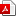# Revisiting the Relationship between β and σ Convergence

### Introduction

The paper questions the widely held assumptions about the relationship between absolute β-convergence and σ-convergence in the existing literature which states that β-convergence is a necessary condition for σ-convergence. The study utilizes the data of 136 countries for the period 1950 to 2008. The non-linear least squares estimations of the β-convergence regression, using both the cross-section and panel data, have confirmed no absolute convergence for the world sample. The σ-convergence has been studied using its two widely used measures, namely, coefficient of variation of income and standard deviation of log income. The results reveal that there is an evidence of σ-convergence using the former measure but not using the latter measure. These findings on β and σ convergence asserts that the relationship between β and σ convergence depends on the particular measure of σ-convergence being used, and hence, cannot be generalized. Specifically, contrary to the theoretical view of relationship between β and σ convergence, there is an evidence of σ-convergence without prevalence of β-convergence among the world countries.VIEW PDF